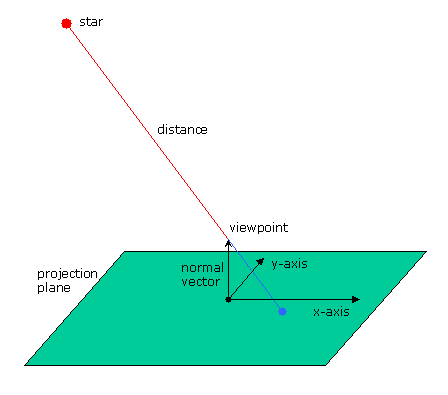introduction screen shots samples old samples physics news download technics planning contact site map
 Physical Modeling

Here the physical and mathematical background of the first simulation are sketched.

## Star distribution

What is the initial star distribution? The first simple approch is a homogen (but random) distribution on a three dimensional sphere. All stars are of equal mass and brightness.

## Projection

To get an visual impression we must choose a viewpoint. We also have to decide about our viewing direction and orientation. These informations fix the position of our virtual photo plate. Now each star position is mapped through the viewpoint onto the photo plate.## Brightness

The brightness of the star makes an little spot on our virtual photo plate. The brightness of the spot depends only from the distance between star and viewpoint. The photo plate is a pixel matrix, that has some implications:

• one pixel could only have a small range of brightness (e.g. between 0 and 255)
• different stars could be mapped onto the same pixel

## Movement of viewpoint

Currently the viewpoint simply circles around the middle of the sphere.

## Movement of stars

The stars will have to move according to Newton's gravitional law. Because there is no analytical solution for the differential calculus problem, we have to use approximations. With a small time unit we calculate the star movements. We have to be carefull, that the stars don't get to close to each other. If this happens the calculation error has a great impact on our dynamical system. To renormalize the system we use the conservational laws of energy and impulse.

Currently we are not carefull. We just use Euler's method to calculate star positions and velocities. The following formulas are used:

`star.velocity[i] = star.velocity[i] - deltat * gamma * sum(j, star[j].mass * (star[i].position - star[j].position) / |(star[i].position - star[j].position| ^ 3)`

`star[i].position = star[i].position[k] + deltat * star.velocity[i]`

This is a very simple single step method. Some more information about better approximation methods to solve the N-body problem can be found at Bob Jenkins' web site and parallel N-body simulations.

update 2006-03-10 02:55:25+0100